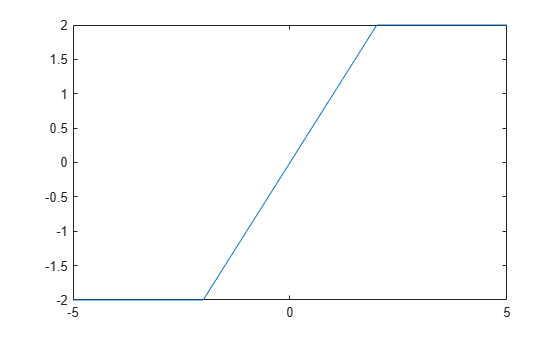# piecewise

Conditionally defined expression or function

## Syntax

``pw = piecewise(cond1,val1,cond2,val2,...)``
``pw = piecewise(cond1,val1,cond2,val2,...,otherwiseVal)``

## Description

example

````pw = piecewise(cond1,val1,cond2,val2,...)` returns the piecewise expression or function `pw` whose value is `val1` when condition `cond1` is true, is `val2` when `cond2` is true, and so on. If no condition is true, the value of `pw` is `NaN`.```

example

````pw = piecewise(cond1,val1,cond2,val2,...,otherwiseVal)` returns the piecewise expression or function `pw` that has the value `otherwiseVal` if no condition is true.```

## Examples

collapse all

Define the following piecewise expression by using `piecewise`.

`$\mathit{y}=\left\{\begin{array}{ll}-1& \mathit{x}<0\\ 1& \mathit{x}>0\end{array}$`

```syms x y = piecewise(x < 0,-1,x > 0,1)```
```y =  ```

Evaluate `y` at `-2`, `0`, and `2` by using `subs` to substitute for `x`. Because `y` is undefined at `x = 0`, the value is `NaN`.

`subs(y,x,[-2 0 2])`
`ans = $\left(\begin{array}{ccc}-1& \mathrm{NaN}& 1\end{array}\right)$`

Define the following function symbolically.

`$\mathit{y}\left(\mathit{x}\right)=\left\{\begin{array}{ll}-1& \mathit{x}<0\\ 1& \mathit{x}>0\end{array}$`

```syms y(x) y(x) = piecewise(x < 0,-1,x > 0,1)```
```y(x) =  ```

Because `y(x)` is a symbolic function, you can directly evaluate it for values of `x`. Evaluate `y(x)` at `-2`, `0`, and `2`. Because `y(x)` is undefined at `x = 0`, the value is `NaN`. For details, see Create Symbolic Functions.

`y([-2 0 2])`
`ans = $\left(\begin{array}{ccc}-1& \mathrm{NaN}& 1\end{array}\right)$`

Set the value of a piecewise function when no condition is true (called otherwise value) by specifying an additional input argument. If an additional argument is not specified, the default otherwise value of the function is `NaN`.

Define the piecewise function

`$y=\left\{\begin{array}{cc}-2& x<-2\\ 0& -2`

```syms y(x) y(x) = piecewise(x < -2,-2,(-2 < x) & (x < 0),0,1)```
```y(x) =  ```

Evaluate `y(x)` between `-3` and `1` by generating values of `x` using `linspace`. At `-2` and `0`, `y(x)` evaluates to `1` because the other conditions are not true.

`xvalues = linspace(-3,1,5)`
```xvalues = 1×5 -3 -2 -1 0 1 ```
`yvalues = y(xvalues)`
`yvalues = $\left(\begin{array}{ccccc}-2& 1& 0& 1& 1\end{array}\right)$`

Plot the following piecewise expression by using `fplot`.

`$y=\left\{\begin{array}{cc}-2& x<-2\\ x& -22\end{array}.$`

```syms x y = piecewise(x < -2,-2,-2 < x < 2,x,x > 2,2); fplot(y)```On creation, a piecewise expression applies existing assumptions. Apply assumptions set after creating the piecewise expression by using `simplify` on the expression.

Assume `x > 0`. Then define a piecewise expression with the same condition `x > 0`. `piecewise` automatically applies the assumption to simplify the condition.

```syms x assume(x > 0) pw = piecewise(x < 0,-1,x > 0,1)```
`pw = $1$`

Clear the assumption on `x` for further computations.

`assume(x,'clear')`

Create a piecewise expression `pw` with the condition `x > 0`. Then set the assumption that `x > 0`. Apply the assumption to `pw` by using `simplify`.

```pw = piecewise(x < 0,-1,x > 0,1); assume(x > 0) pw = simplify(pw)```
`pw = $1$`

Clear the assumption on `x` for further computations.

`assume(x,'clear')`

Differentiate, integrate, and find limits of a piecewise expression by using `diff`, `int`, and `limit` respectively.

Differentiate the following piecewise expression by using `diff`.

`$\mathit{y}=\left\{\begin{array}{ll}1/\mathit{x}& \mathit{x}<-1\\ \mathrm{sin}\left(\mathit{x}\right)/\mathit{x}& \mathit{x}\ge -1\end{array}$`

```syms x y = piecewise(x < -1,1/x,x >= -1,sin(x)/x); diffy = diff(y,x)```
```diffy =  ```

Integrate `y` by using `int`.

`inty = int(y,x)`
```inty =  ```

Find the limits of `y` at `0` by using `limit`.

`limit(y,x,0)`
`ans = $1$`

Find the right- and left-sided limits of `y` at `-1`. For details, see `limit`.

`limit(y,x,-1,'right')`
`ans = $\mathrm{sin}\left(1\right)$`
`limit(y,x,-1,'left')`
`ans = $-1$`

Add, subtract, divide, and multiply two piecewise expressions. The resulting piecewise expression is only defined where the initial piecewise expressions are defined.

```syms x pw1 = piecewise(x < -1,-1,x >= -1,1); pw2 = piecewise(x < 0,-2,x >= 0,2); add = pw1+pw2```
```add =  ```
`sub = pw1-pw2`
```sub =  ```
`mul = pw1*pw2`
```mul =  ```
`div = pw1/pw2`
```div =  ```

Modify a piecewise expression by replacing part of the expression using `subs`. Extend a piecewise expression by specifying the expression as the otherwise value of a new piecewise expression. This action combines the two piecewise expressions. `piecewise` does not check for overlapping or conflicting conditions. Instead, like an if-else ladder, `piecewise` returns the value for the first true condition.

Change the condition `x < 2` in a piecewise expression to `x < 0` by using `subs`.

```syms x pw = piecewise(x < 2,-1,x > 0,1); pw = subs(pw,x < 2,x < 0)```
```pw =  ```

Add the condition `x > 5` with the value `1/x` to `pw` by creating a new piecewise expression with `pw` as the otherwise value.

`pw = piecewise(x > 5,1/x,pw)`
```pw =  ```

## Input Arguments

collapse all

Condition, specified as a symbolic condition or variable. A symbolic variable represents an unknown condition.

Example: x > 2

Value when condition is satisfied, specified as a number, vector, matrix, or multidimensional array, or as a symbolic number, variable, vector, matrix, multidimensional array, function, or expression.

Value if no conditions are true, specified as a number, vector, matrix, or multidimensional array, or as a symbolic number, variable, vector, matrix, multidimensional array, function, or expression. If `otherwiseVal` is not specified, its value is `NaN`.

## Output Arguments

collapse all

Piecewise expression or function, returned as a symbolic expression or function. The value of `pw` is the value `val` of the first condition `cond` that is true. To find the value of `pw`, use `subs` to substitute for variables in `pw`.

## Tips

• `piecewise` does not check for overlapping or conflicting conditions. A piecewise expression returns the value of the first true condition and disregards any following true expressions. Thus, `piecewise` mimics an if-else ladder.

## Version History

Introduced in R2016b